# A Comprehensive Guide to NumPy Data Types

NumPy, one of the most popular Python libraries for both data science and scientific computing, is pretty omnivorous when it comes to data types.

It has its own set of 'native' types which it is capable of processing at full speed, but it can also work with pretty much anything known to Python.

The article consists of seven parts:

## Integers

The integer types table in NumPy is trivial for anyone with minimal experience in C/C++:

Just like in C/C++, 'u' stands for 'unsigned' and the digits represent the number of bits used to store the variable in memory (eg np.int64 is an 8-bytes-wide signed integer).

When you feed a Python int into NumPy, it gets converted into a native NumPy type called np.int32 (or np.int64 depending on the OS, Python version, and the magnitude of the initializers):

``````>>> import numpy as np
>>> np.array([1, 2, 3]).dtype
dtype('int64')        # int32 on Windows, int64 on Linux and MacOS
``````

If you're unhappy with the flavor of the integer type that NumPy has chosen for you, you can specify one explicitly via the 'dtype' (=data type) argument which accepts either a dtype object `np.array([1,2,3], np.uint8)` or a string `np.array([1,2,3], 'uint8')`.

Why would you want the non-default dtype? Consider the following example (on Windows):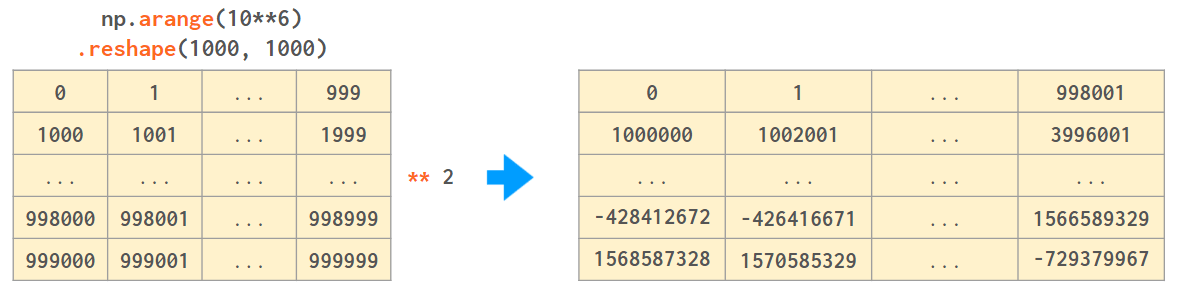What's going on here?

NumPy works best when the width of the array elements is fixed. It is faster and takes less memory, but unlike an ordinary Python int (that works in arbitrary precision arithmetic), the values of an array will wrap when they cross the maximum (or minimum) value for the corresponding data type: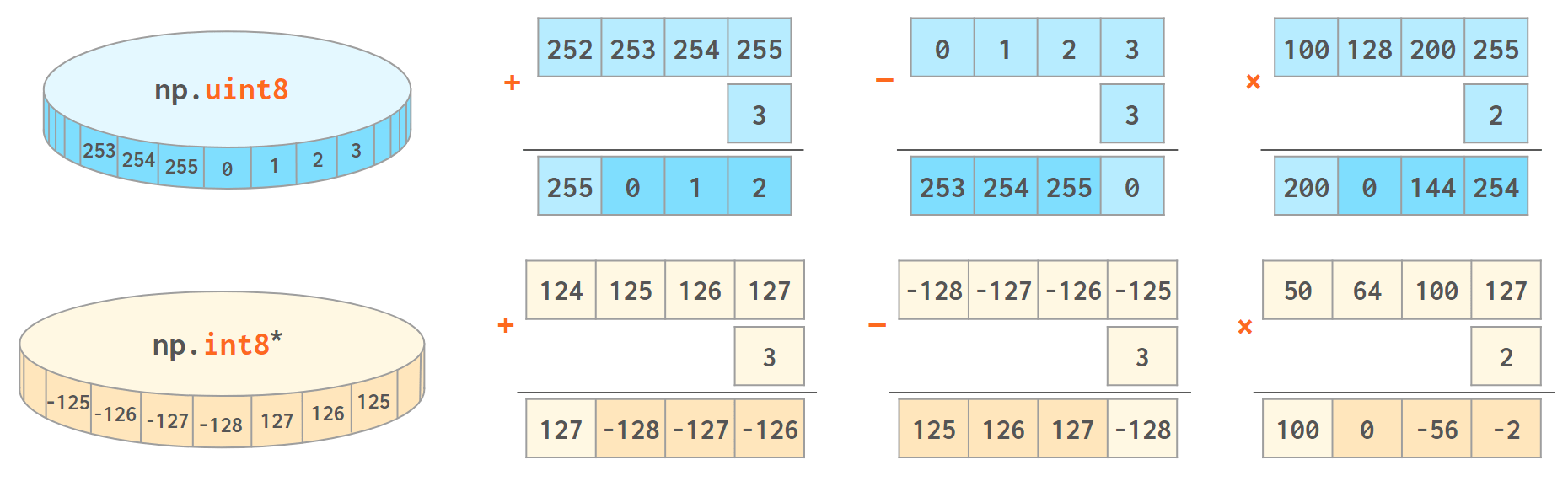What's going on here?

With scalars, it is a different story: first NumPy tries its best to promote the value to a wider type, then, if there is none, fires the overflow warning (to avoid flooding the output with warnings — only once):

The reasoning behind such a discrimination is like this:

You can turn it into an error:NumPy overflow error

or suppress it temporarily: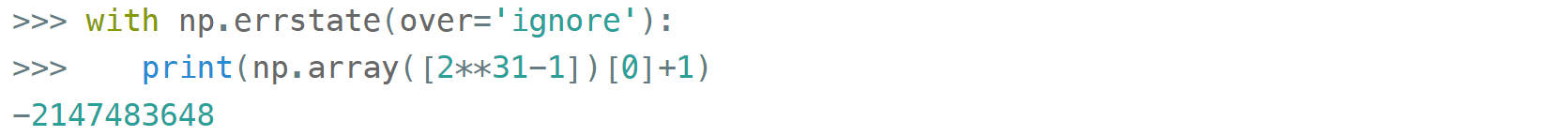NumPy overflow error

Or completely: `np.warnings.filterwarnings('ignore', 'overflow')`

But you can't expect it to be detected when dealing with any arrays. Back to our example, the right way of doing it is to specify the proper dtype:On Linux/MacOS it is np.int64 by default

NumPy also has a bunch of C-style aliases (eg. np.byte is np.int8, np.short is np.int16, np.intc is an int with whichever width int type has in C, etc), but they are getting gradually phased out (eg deprecation of np.long in NumPy v1.20.0) as 'explicit is better than implicit' (but see a present-day usage of `np.longdouble` below).

And yet some more exotic aliases:

• np.int_ is np.int32 on 64bit Windows but np.int64 on 64bit Linux/MacOS, used to designate the 'default' int. Specifying `np.int_` (or just int) as a dtype means 'do what you would do if I didn't specify any dtype at all': `np.array([1,2])`, `np.array([1,2], np.int_)` and `np.array([1,2], int)` are all the same thing.

• np.intp is np.int32 on 32bit Python but np.int64 on 64bit Python, `≈ssize_t` in C, used in Cython as a type for pointers.

Occasionally it happens that some values in the array display anomalous behavior or missing, and you want to process the array without deleting them (eg there's some valid data in other columns).

You can't put `None` there because it doesn't fit in the consecutive np.int64 values and also because `1 + None` is an unsupported operation.

Pandas has a separate data type for that, but NumPy's way of dealing with the missed values is through the so-called masked array: you mark the invalid values with a boolean mask and then all the operations are carried out as if the values are not there.

Finally, if for some reason you need arbitrary-precision integers (Python ints) in ndarrays, numpy is capable of doing it, too:

— but without the usual speedup as it will store references instead of the numbers themselves, keep boxing/unboxing Python objects when processing, etc.

## Floats

As pure Python float did not diverge from the IEEE 754-standardized C double type (note the difference in naming), the floating point numbers transition from Python to NumPy is pretty much hassle-free: Python float is directly compatible with np.float64 and Python complex — with np.complex128.

• As reported by `np.finfo(np.float<nn>).precision`. Two alternative definitions give 15 and 17 digits for np.float64, 6 and 9 for np.float32, etc.

Like integers, floats are also subject to overflow errors.

Suppose you're calculating a sigmoid activation function of the array and one of its elements happens to be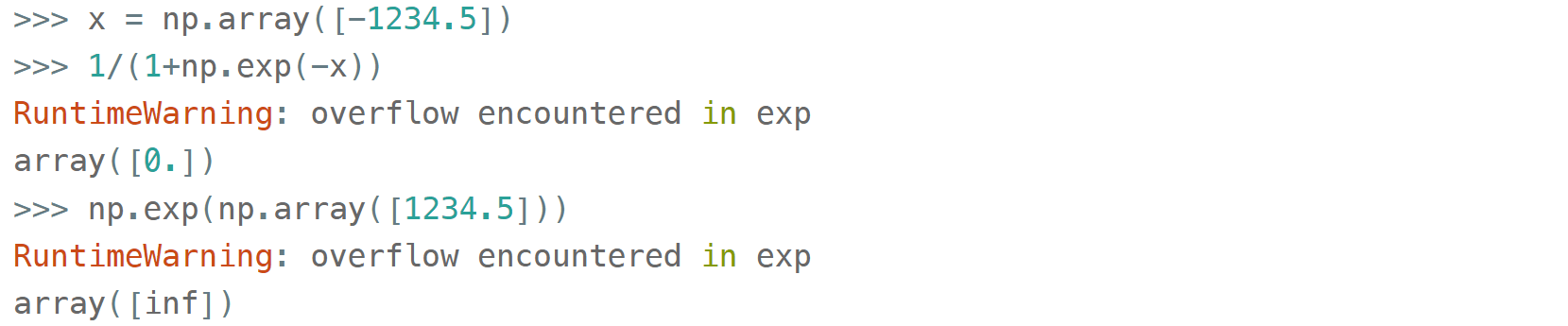NumPy float overflow

What this warning is trying to tell you is that NumPy is aware that mathematically speaking `1/(1+exp(-x))` can never be zero, but in this particular case due an overflow it is.

Such warnings can be 'promoted' to exceptions or silenced via the errstate or filterwarnings as described in the 'integers' section above — and maybe for this particular case that would be enough — but if you really want to get the exact value you can select a wider dtype:

One thing that distinguishes floats from integers is that they are inexact. You can't compare two floats with `a == b`, unless you're sure they are represented exactly. You can expect floats to exactly represent integers — but only below a certain level (limited by the number of the significant digits):

Also exactly representable are fractions like 0.5, 0.125, 0.875 where the denominator is a power of 2 (0.5=1/2, 0.125=1/8, 0.875 =7/8, etc). Any other denominator will result in a rounding error so that 0.1+0.2!=0.3.

The standard approach of dealing with this problem (as well as with source #2 of inexactness: rounding of the results of the calculations) is to compare them with a relative tolerance (to compare two non-zero arguments) and absolute tolerance (if one of the arguments is zero). For scalars, it is handled by `math.isclose(a, b, *, rel_tol=1e-09, abs_tol=0.0)`, for NumPy arrays there's a vector version `np.isclose(a, b, rtol=1e-05, atol=1e-08)`. Note that the tolerances have different names and defaults.

For the financial data decimal.Decimal type is handy as it involves no additional tolerances at all:

But it is not a silver bullet: it also has rounding errors (see source #2 above). The only problem it solves is the exact representation of decimal numbers that humans are used to.

Plus it doesn't support anything more complicated than arithmetic operations (though logarithm and square root are supported) and runs slower than floats.

For pure mathematical calculations fractions.Fraction can be used: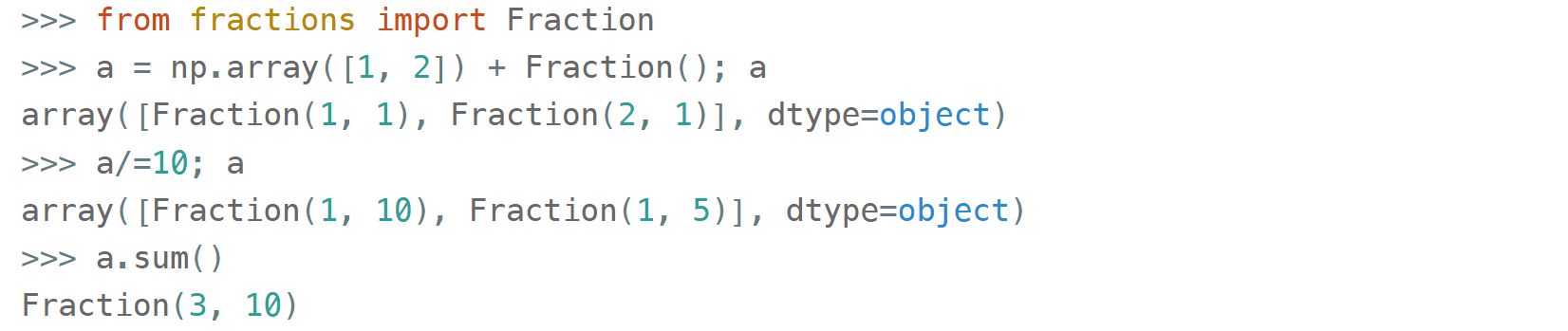Python fractions module

It can exactly represent any rational numbers and is not subject to rounding errors during the calculations (source #2) but π and e are out of luck! If you need them, too, then SymPy is your friend.

Both Decimal and Fraction are not native types for NumPy but it is capable of working with them with all the niceties like multi-dimensions and fancy indexing, albeit at the cost of slower processing speed than that of native ints or floats.

Complex numbers are treated the same way as floats. There are extra convenience functions with intuitive names like np.real(z), np.imag(z), np.abs(z), np.angle(z) that work on both scalars and arrays as a whole. The only difference from the pure Python `complex`, `np.complex_` does not work with integers:

Just like with the integers, in float (and complex) arrays it is also sometimes useful to treat certain values as 'missing'. Floats are better suited for storing anomalous data: they have a math.nan (or `np.nan` or `float('nan'))` value which can be stored inline with the 'valid' numeric values.

But nan is contagious in the sense that all the arithmetic with nan results in nan. Most common statistical functions have a nan-resistant version (np.nansum, np.nanstd, etc), but other operations on that column or array would require prefiltering. Masked arrays automate this step: the mask can only be built once, then it is 'glued' to the original array so that all subsequent operations only see the unmasked values and operate on them.

Also the names `float96` / `float128` are somewhat misleading. Under the hood it is not `__float128` but whichever `longdouble` means in the local C++ flavor. On x86_64 Linux it is float80 (padded with zeros for memory alignment) which is certainly wider than `float64`, but it comes at the cost of the processing speed. Also you risk losing precision if you inadvertently convert to Python float type. For better portability it is recommended to use an alias np.longdouble instead of `np.float96` / `np.float128` because that's what will be used internally anyway.

More insights on floats can be found in the following sources:

## Bools

The boolean values are stored as single bytes for better performance. np.bool_ is a separate type from Python's bool because it doesn't need reference counting and a link to the base class required for any pure Python type. So if you think that using 8 bits to store one bit of information is excessive look at this:

`np.bool` is 28 times more memory efficient than Python's bool — though in real-world scenarios the rate is lower: when you pack NumPy bools into an array, they will take 1 byte each, but if you pack Python bools into a list it will reference the same two values every time, costing effectively 8 bytes per element on x86_64:

The underlines in `bool_`, `int_`, etc are there to avoid clashes with Python's types. It's a bad idea to use reserved keywords for other things, but in this case it has an additional advantage of allowing (a generally discouraged, but useful in rare cases) `from numpy import *` without shadowing Python bools, ints, etc. As of today, `np.bool` still works but displays a deprecation warning.

## Strings

Initializing a NumPy array with a list of Python strings packs them into a fixed-width native NumPy dtype called np.str_. Reserving a space necessary to fit the longest string for every element might look wasteful (especially in the fixed USC-4 encoding as opposed to 'dynamic' choice of the UTF width in Python str)

The abbreviation <U4 comes from the so-called array protocol introduced in 2005. It means little-endian USC-4-encoded string, 5 elements long (USC-4≈UTF-32, a fixed width, 4-bytes per character encoding). Every NumPy type has an abbreviation — as unreadable as this one — luckily have they adopted human-readable names at least for the most used dtypes.

Another option is to keep references to Python strs in a NumPy array of objects:

The first array memory footprint amounts to 164 bytes, the second one takes 128 bytes for the array itself + 154 bytes for the three Python `str`s:

Depending on the relative lengths of the strings and the number of the repeated string either one approach can be a significant win or the other.

If you're dealing with a raw sequence of bytes NumPy has a fixed-length version of a Python bytes type called np.bytes_:

Here `|S5` means endianness-unappliable sequence of bytes 5 elements long'.

Once again, an alternative is to store the Python bytes in the NumPy array of objects:

This time the first array takes 124 bytes, the second one is the same 128 bytes for the array itself + 106 bytes for the three Python `bytes`:

We see that `str_` is smaller again, yet for more diverse lengths `str` can take the win.

As for the native np.str_ and np.bytes_ types, NumPy has a handful of common string operations. They mirror Python's str methods, live in the np.char module and operate over the whole array:

With object-mode strings the loops must happen on the Python level:

According to my benchmarks, basic operations work somewhat faster with `str` than with `np.str_`.

## Datetimes

NumPy introduces an interesting native data type for datetimes, similar to a POSIX timestamp (aka Unix time, the number of seconds since 1 Jan 1970) but capable of counting time with a configurable granularity — from years to attoseconds — represented invariably by a single `int64` number.

• Years granularity means just count the years — no real improvement against storing years as an integer.
• Days granularity is an equivalent of Python's datetime.date
• Microseconds — of Python's datetime.datetime

And everything below is unique to np.datetime64.

When creating an instance of `np.datetime64`, NumPy chooses the most coarse granularity that can still hold such data:

When creating an array you decide if you are ok with the granularity that NumPy has chosen for you or you insist on, say, nanoseconds or what not, and it'll give you 2⁶³ equidistant moments measured in the corresponding units of time to either side of 1 Jan 1970.

It is possible to have a multiple of a base unit. For example, if you only need a precision of 0.1 sec, you don't necessarily need to store milliseconds:

To get a machine-readable representation of the granularity without parsing the dtype string:

Just like in pure Python when you subtract one `np.datetime64` from another you get a `np.timedelta64` object (also represented as a single int64 with a configurable granularity). For example, to get the number of seconds until the New Year,

Or if you don't care about the fractional part, simply

Once constructed there's not much you can do about the datetime or timedelta objects. For the sake of speed, the amount of available operations is kept to the bare minimum: only conversions and basic arithmetic. For example, there are no 'years' or 'days' helper methods.

To get a particular field from a datetime64/timedelta64 scalar you can convert it to a conventional datetime:

For the arrays like this one

you can either make conversions between np.datetime64 subtypes (faster)

or use Pandas (2-4 times slower):

Here's a useful function that decomposes a `datetime64` array to an array of 7 integer columns (years, months, days, hours, minutes, seconds, microseconds):

A couple of gotchas with datetimes:

leap seconds (an essential part of both UTC and ordinary wall time) are not:

To be fair, neither `datetime.datetime` nor even `pytz` counts them, either (although in general it is possible to extract info about them with pytz). time module supports them only formally (accepts 60th second, but gives incorrect intervals).

It looks as if only astropy processes them correctly so far,

others adhere to the proleptic Gregorian calendar with its exactly 86400 SI seconds a day that has already gained about half a minute difference with the wall time since 1970 due to irregularities of the Earth rotation.

The practical implications of using this calendar are:

• mistake when calculating intervals that include one or more leap seconds
• exception when trying to construct a datetime64 from a timestamp taken during a leap second

Finally, note that all the times in `np.datetime64` are 'naive': they are not 'aware' of daylight saving (so it is recommended to store all datetimes in UTC) and are not capable of being converted from one timezone to another (use pytz for timezone conversions):

## Combinations thereof

A 'structured array' in NumPy is an array with a custom `dtype` made from the types described above as the basic building blocks (akin to `struct` in C). A typical example is an RGB pixel color: a 3 bytes long type (usually 4 for alignment), in which the colors can be accessed by name:

To be able to access the fields as attributes, a np.recarray can be used:

Here it works like `reinterpret_cast` in C++, but sure enough, recarray can be created on its own, without being a view of something else.

Types for the structured arrays do not necessarily need to be homogeneous and can even include subarrays.

With structured arrays and recarrays can get the 'look and feel' of a basic Pandas DataFrame:

• you can address columns by names
• do some arithmetic and statistic calculations with them
• you can handle missing values efficiently
• some operations are faster in NumPy than in Pandas

But they lack:

• grouping (except what is offered by `itertools.groupby`)
• the mighty pandas Index and MultiIndex (so no pivot tables)
• other niceties like convenient sorting, etc.

The gotcha here is that even though this syntax is convenient for addressing particular columns as a whole, neither structured arrays nor recarrays are something you'd want to use in the innermost loop of a compute-intensive code:

## Type Checks

One way to check NumPy array type is to run `isinstance` against its element:

All the NumPy types are interconnected in an inheritance tree displayed at the top of the article (blue=abstract classes, green=numeric types, yellow=others) so instead of specifying a whole list of types like `isinstance(v, [np.int32, np.int64, etc])` you can write more compact type checks like

The downside of this method is that it only works against a `value of the array`, not against the `array itself`. Which is not useful when the array is empty, for example. Checking the type of the array is more tricky.

For basic types the `==` operator does the job for a single type-check:

and `in` operator for checking against a group of types:

But for more sophisticated types like `np.str_` or `np.datetime64` they don't.

The recommended way⁴ of checking the dtype against the abstract types is

It works with all native NumPy types, but the necessity of this method looks somewhat non-obvious: what's wrong with good old `isinstance`? Obviously, the complexity of `dtypes` inheritance structure (they are constructed 'on the fly'!) didn't allow them to do it according to the principle of least astonishment.

If you have Pandas installed, its type checking tools work with NumPy dtypes, too:

Yet another method is to use (undocumented, but used in SciPy/NumPy codebases, eg here) `np.typecodes` dictionary. The tree it represents is way less branchy:

Its primary application is to generate arrays with specific dtypes for testing purposes, but it can also be used to distinguish between different groups of dtypes:

One downside of this method is that bools, strings, bytes, objects, and voids ('?', 'U', 'S', 'O', and 'V', respectively) don't have dedicated keys in the dict.

This approach looks more hackish yet less magical than `issubdtype`.

I would like to thank members of the NumPy team for their help in chasing the typos and for the productive discussion of some advanced concepts.

1. Ricky Reusser, Half-Precision Floating-Point, Visualized

2. Floating point guide https://floating-point-gui.de/

3. David Goldberg, What Every Computer Scientist Should Know About Floating-Point Arithmetic, Appendix D

4. NumPy issue #17325, Add a canonical way to determine if dtype is integer, floating point or complex.